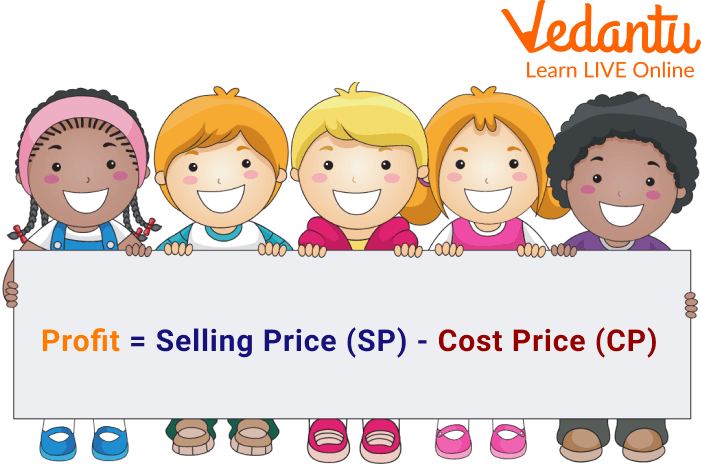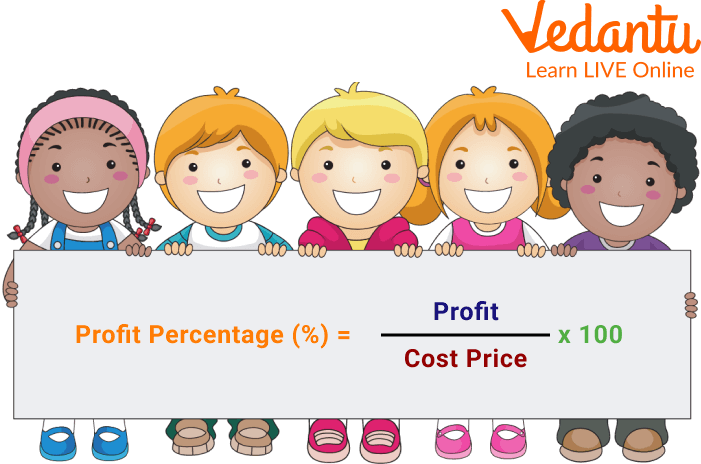Courses
Courses for Kids
Free study material
Free LIVE classes
More

# Profit Percent: What it is and its ExamplesLIVE
Join Vedantu’s FREE Mastercalss

## Introduction to Profit Percent

In mathematics, the gain from any business operation is referred to as profit. Every time a shopkeeper sells a product, he aims to profit by getting something from the customer. In other words, if he sells the goods for more than their cost price, he makes a profit; yet, if he needs to sell them for less, he loses money. With the help of formulae and examples, let's study how to calculate profit and profit per cent in this article.

## What Do You Mean by Profits?

Generally, a product's profit is defined as the sum received from sales, which should exceed the product's cost price. It is the gain from any type of commercial activity. In other words, if a product's selling price (SP) is higher than its cost price (CP), there has been a gain or profit.

## Profit Formula

The profit formula helps calculate the profit in any financial transaction and the profit made by selling a specific product, typically done in a business. A profit can be determined when the selling price exceeds the cost price. So, the method to calculate the profit is:

Profit = Selling Price (S.P.) - Cost Price (C.P.)

Where

The Cost Price of the product is the cost at which it was originally bought.

The Selling Price of the product is the cost at which it was sold.Profit Formula

## Profit Percentage

Profit percentage (%) is the amount of profit expressed in terms of percentage. This profit is based on the cost price. Hence, the formula to find the profit percentage is

$\text { Profit Percentage }(\%)=\dfrac{\text { Profit }}{\text { Cost Price }} \times 100$

## Formula for Profit PercentageFormula for Profit Percentage

## Calculate Profit and Profit Percent

• Identify the selling price of the products at cost.

• Next, determine the total selling price of the purchased items.

• To calculate your profit, deduct the cost and selling prices.

• Divide the profit amount by the cost price to determine the profit margin.

• To convert the profit margin to a percentage, multiply it by 100.

Note: We can also use a percentage profit calculator to find the profit and profit percentage.

Let’s see one profit and profit percent example: A shopkeeper buys a watch in bulk for Rs. 20 each. He sells them for Rs. 45 each. Calculate the profit and the profit percentage.

Ans: Given,

Selling price of the watch = Rs. 45

Cost price of the watch = Rs. 20.

Now, Profit = Selling Price – Cost Price

So, the profit on the watch = 45 – 20 = Rs. 25.

Therefore, the profit on the watch will be of Rs. 25.

Using the formula for profit percentage,

$\text { Profit Percentage }(\%)=\dfrac{\text { Profit }}{\text { Cost Price }} \times 100$

So, the profit percentage of the shopkeeper will be

= $\dfrac{25}{20} \times 100$

=125%

It can be said that the shopkeeper made a profit of Rs. 25 from each watch with a profit percentage of 125%.

## Percentage Profit Solved Problems

Q 1. Ram buys an old scooter for Rs. 4700 and spends Rs. 800 on its repairs. If he sells the scooter for Rs. 5800, his gain percent is:

Ans:

Cost Price (C.P.) = Rs. (4700 + 800) = Rs. 5500.

Selling Price (S.P.) = Rs. 5800.

Profit = Selling Price (S.P.) - Cost Price (C.P.)

Profit = (S.P.) - (C.P.) = Rs.(5800 - 5500) = Rs. 300.

$\text { Profit Percentage }(\%)=\dfrac{\text { Profit }}{\text { Cost Price }} \times 100$

Profit % = $\dfrac{300}{5500} \times 100$

= 5.45%

Q 2. If the cost of 18 mangoes is the same as the selling price of 16 mangoes. Find the gain percent.

Ans: Let the cost price of each mango be re.1

Then,

C.P. of 18 mangoes= Rs. 18

S.P. of 16 mangoes= Rs. 18

Now,

C.P. of 16 mangoes= Rs. 16

Profit= S.P. - C.P.

= Rs. (18-16)= Rs. 2

$\text { Profit Percentage }(\%)=\dfrac{\text { Profit }}{\text { Cost Price }} \times 100$

Profit % = $\dfrac{2}{16} \times 100$

= 12.5%

## Practice Problems

Q 1. If a store owner sells mango for 200 rupees per kg, their cost is 150 rupees per kg. Then determine the shop owner's profit. (Ans: Rs. 50)

Q 2. Ram made a 25% profit when he sold a watch for Rs. 5,000. How much does the camera cost? (Rs. 4000)

## Summary

In this article, we have learned that the revenue generated from selling a good or anything is referred to as the profit, and it must exceed the cost of the good. In other terms, a gain from any business activity constitutes a profit. Hence, the profit is the difference between the selling and cost prices. Later, we have seen the profit percentage. i.e. $\text { Profit Percentage }(\%)=\dfrac{\text { Profit }}{\text { Cost Price }} \times 100$. So by using these two, one can know how much gain they have made while purchasing or selling goods. In the end, we added some solved examples and practice problems to clarify the topic's understanding.

Last updated date: 29th Sep 2023
Total views: 68.1k
Views today: 0.68k

## FAQs on Profit Percent: What it is and its Examples

1. Why is profit and loss important in any business?

The profit and loss account details an enterprise's income and expenses, which result in net profit or a net loss. It assists a businessman in evaluating an enterprise's performance and provides a foundation for forecasting future performance.

2. Does turnover signify revenue or profit?

Turnover and profit are not the same things in business. The phrase "revenue" refers to all business earnings over a specific time period. Profit is the gain realized after deducting all costs associated with conducting business.

3. What is the Gross Profit Formula?

A company's profit after deducting its manufacturing and selling expenses from its total sales is known as gross profit. The gross profit is determined using the formula: gross profit = total sales (revenue) - the cost of goods sold.